# How to use the COUNTIF function in ExcelA common task in Excel often involves taking a set of data and tallying the number of occurrences that match certain characteristics. The COUNTIF Excel function allows you to do just that: it counts the number of data points (cells) that meet a certain criteria that you specify within the function.

Because of the wide range of criteria that you can input, the COUNTIF function allows you to filter your data in virtually any way you want.

### Syntax

=COUNTIF(range, criteria)
• range is the group of cells you wish to count
• criteria is the characteristic that will decide whether or not each cell gets counted. This can be an expression, number, cell reference, or text string.

### How to use COUNTIF in Excel

#### Criteria as text string

To illustrate some of the ways that you can use the COUNTIF function in Excel, take a look at the dataset below. It outlines the dietary preferences of a group of people. In the first example, I use COUNTIF to tally the number of vegetarians in the group by typing =COUNTIF(D2:D9,”Yes”).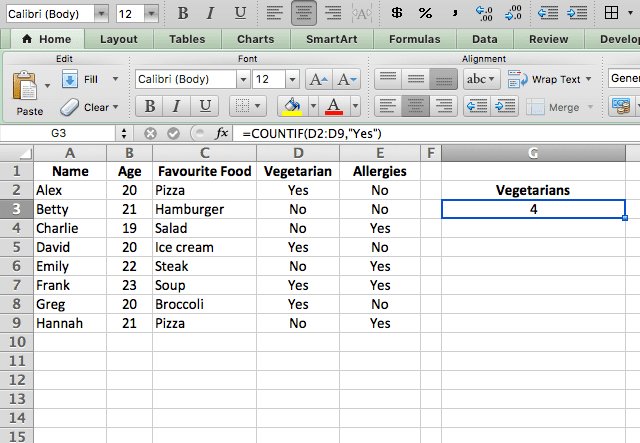In this example, the criterion that I’ve specified is a text string. The function looks at all the cells in the range that I’ve indicated, and returns the number of cells that match the criteria – in this case, the number of instances where “Yes” appears in the column.

#### Criteria as cell reference

Another way of indicating the criteria is to use a cell reference. Take a look at the example below. Here, I count the number of people with allergies by using cell E4 as my criteria. Of course, that cell contains the text string Yes, so this is just an alternative to typing “Yes” into the formula.

The formula I use here is =COUNTIF(E2:E9,E4).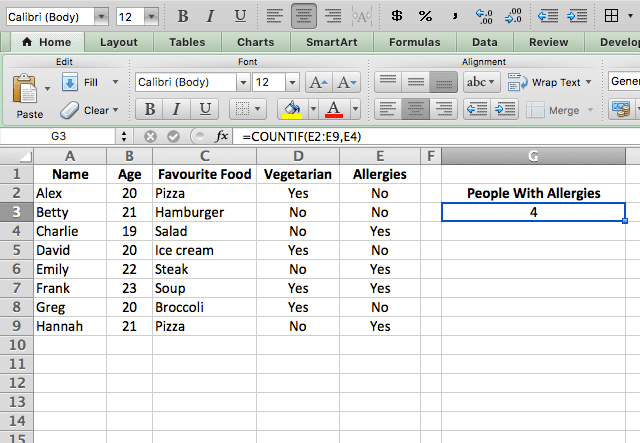#### Criteria as a number

You can also put a number as your criteria in the COUNTIF function. In this example, I want to count only people of a certain age, so I put the age directly into the function.

Notice that unlike a text string,  you don’t need to put quotation marks around a number in a formula.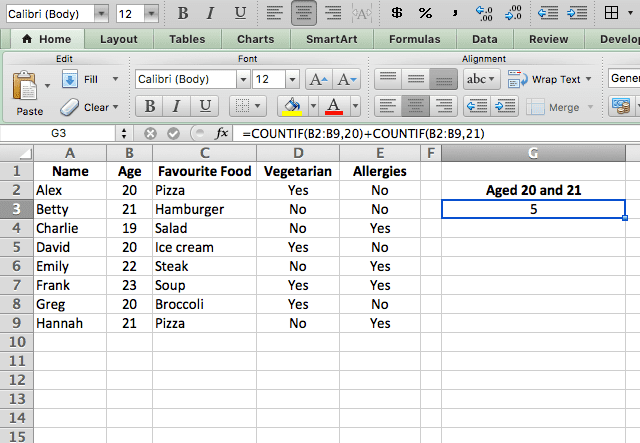Another thing I’ve done differently here is: I added two COUNTIF functions together. The reason for this is is that I want to count all the people aged 20 and 21.

In order to do this, I use two separate COUNTIF functions. This may sound cumbersome, but the good news is that because COUNTIF just gives a final tally of cells that meet the criteria, you can use multiple COUNTIF functions in the same formula. You simply add them together to get the total count of all cells that match multiple criteria. You can also subtract COUNTIF functions when you want to get cells that are between two criteria.

There’s another way to use multiple criteria – I suggest you look into the COUNTIFS function if you’re interested in this!

#### Criteria with logical operator

Logical operators such as > and < (greater than & less than) can be used within your criteria.

In the example below, I use the < symbol to count all the people younger than 23 in my dataset, by typing =COUNTIF(B2:B9,”<23″).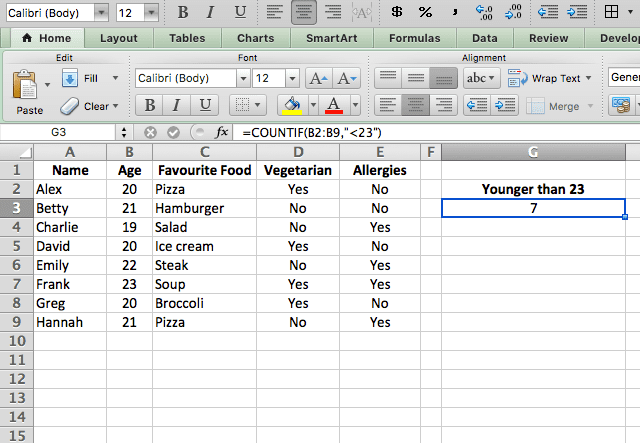Note that this criteria includes both the logical operator symbol and a number. The criteria must be enclosed in quotation marks, unlike when you use a number only.

The following logical operators can be used in the same way: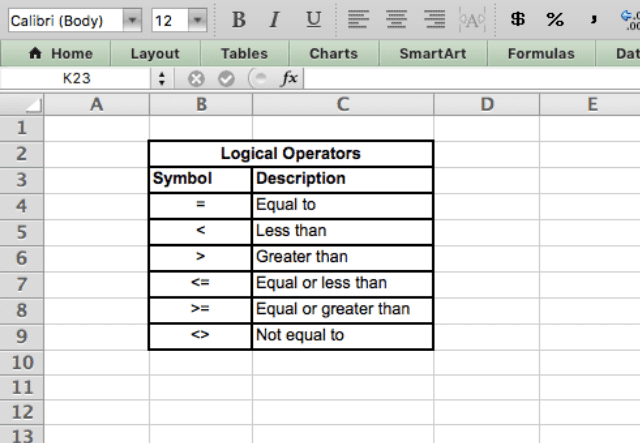#### Concatenated criteria

You’ve now seen the criteria part of the function as a text string, a number, and a cell reference.

Let me show you how to do a combination of these by concatenating (combining) different criteria using the ampersand (&). I do this below by typing =COUNTIF(B2:B9,”<“&B7).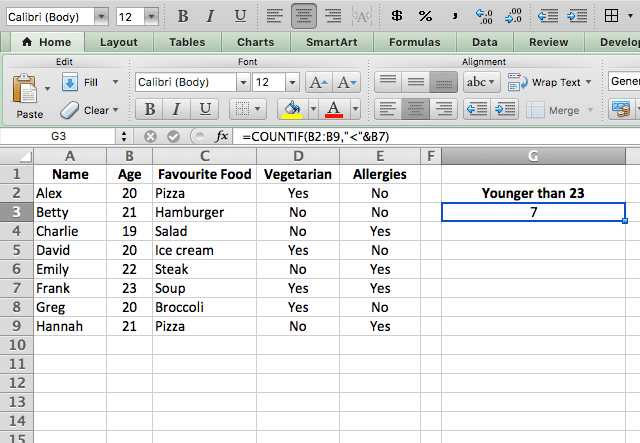The result of this function is exactly the same as the previous example.

Except, I have now used the & symbol to concatenate a cell reference (B7) which contains the number 23, instead of simply typing the number 23 into the formula.

#### Wildcards

On top of the different ways to set the criteria in the COUNTIF function, there is something else called “wildcards”.

The wildcard characters * and ? can be used within your criteria to indicate a match with any character (?) or any sequence of characters (*).

The example below has very specific criteria. I want to count all the occurrences of a 5-letter name that ends in “y”. To do this, you can use the ? wildcard.

This wildcard character is a stand-in for any character – so when I type =COUNTIF(A2:A9,”????y”) I indicate that I want to count all instances where there are four characters (which can be anything) followed by a “y”.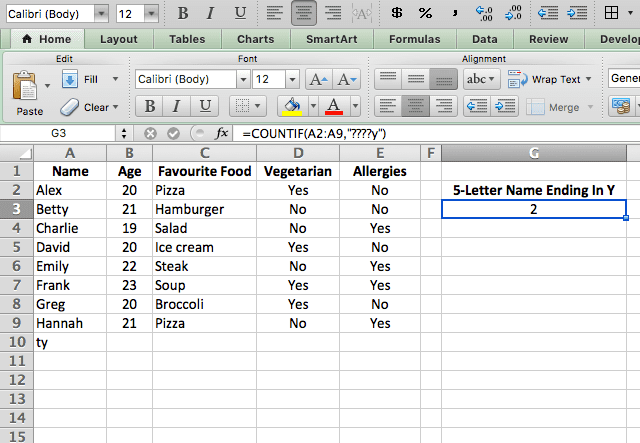Use the second wilcard (*) when the length of the criteria doesn’t matter – for instance, if I didn’t care about the length of the name, just that it ended in “y”, the criteria could be “*y”.

Another handy use for the * wildcard is to get rid of blank cells. I demonstrate that in the screenshot below using the formula =COUNTIF(A2:A13,”*”).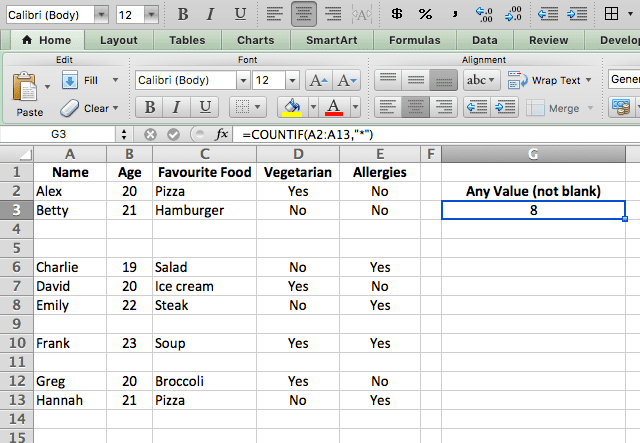Because the * represents any number of any characters, the only cells that don’t meet this criteria are the ones that don’t contain anything at all!

### Countif Excel

By now, you hopefully have a good grasp on the many ways the Excel COUNTIF function can be used, and can put it into practice with your own data. Happy counting!

If you’d like to learn more about the various formulas of Google Sheets, why not take a look at our blog post on the DCOUNT formula in Google Sheets.